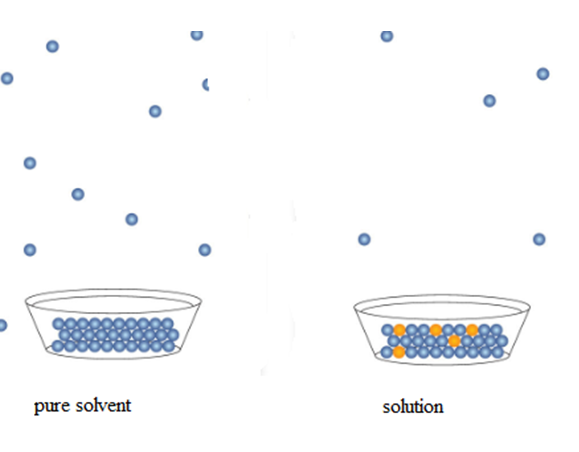# A:Which of the following substances has the highest vapor pressure?Group of answer choices3.0 M NaCl(aq)0.10 M NaCl(aq)water0.25 M NaCl(aq) B:At room temperature, 5.25 g of Ce2(SO4)3 can dissolve in 50.0 g of water.  What is the mole fraction of Ce2(SO4)3 in this solution?Group of answer choices2.780.003310.009240.0950

Question
147 views

A:

Which of the following substances has the highest vapor pressure?

3.0 M NaCl(aq)
0.10 M NaCl(aq)
water
0.25 M NaCl(aq)

B:

At room temperature, 5.25 g of Ce2(SO4)3 can dissolve in 50.0 g of water.  What is the mole fraction of Ce2(SO4)3 in this solution?

2.78
0.00331
0.00924
0.0950
check_circle

Step 1

A)

The pressure exerted by vapor is called vapor pressure.Step 2

Pure solvent has more vapor pressure than its corresponding solution.

As some of the space on the surface of the solvent is occupied by solue molecules, the vapor pressure of pure solvent decreases.

So, if the amount of solute increases in the solution, the vapor pressure of the solution decreases more.

Step 3

In the given data,

3.0M NaCl aqueous solution (solution)

0.10 M NaCl(aq)(solution)

water (pure solvent)

0.25 M NaCl(aq)  (solution)

Among them water is the p...

### Want to see the full answer?

See Solution

#### Want to see this answer and more?

Solutions are written by subject experts who are available 24/7. Questions are typically answered within 1 hour.*

See Solution
*Response times may vary by subject and question.
Tagged in

### Chemistry